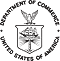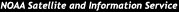formerly the National Oceanographic Data Center (NODC)...  more on NCEIThis Site All of NOAA

NCEI is transitioning to a new website and paths to data resources will be changing. Please contact NCEI.Info@noaa.gov with any questions of issues. See the new website at www.ncei.noaa.gov.

 You are here: Home > Ocean Climate Laboratory > OCL Products > WOA01 > Online Data > One-degree data retrieval table

World Ocean Atlas 2001 (one-degree objectively analyzed fields and statistics)

Note: retrieval software on this site requires a JavaScript compatible browser. For best support, we suggest that you use Mozilla 1.73/Firefox 1.0 and up (download from site*).

This is a searchable WOA01 inventory table. Where: a = annual, s = seasonal, m = monthly.
 One-degree Fields Number of observations Analyzed mean Unanalyzed mean Unanalyzed mean minus analyzed Standard deviation Standard error Grid points Seasonal or monthly minus annual analyzed mean ALL FIELDS Time period Time period Time period Time period Time period Time period Time period Time period Time period Time period Variables a s m a s m a s m a s m a s m a s m a s m s m a s m Temperature Salinity Dissolved oxygen Apparent oxygen utilization Percent oxygen saturation Phosphate Nitrate Silicate Chlorophyll Plankton ALL VARIABLES

Data are organized by variable. Within each variable directory, the data are stored by time period: annual, seasonal, and monthly. Each time period directory contains the following file types:

• Analyzed mean fields (an) - one-degree all-data objectively analyzed mean. For temperature, salinity, and oxygen, an denotes an average of either the 12 monthly analyses (used to compute the annual analysis at and above 1500 meters), the 3 monthly analyses (used for the seasonal analyses), or the 4 seasonal analyses (used for the annual analysis below 1500 meters). For nutrients and chlorophyll data, an denotes the average of the 4 seasonal analyses. For annual at and above 1500 meters, this is the average of the 12 monthly analyses, for annual below 1500 meters, this is the average of the 4 seasonal analyses, for seasonal means this is the average of the appropriate 3 monthly analyses that comprise each season)
• Seasonal or monthly fields minus annual fields (ma) - one-degree seasonal and monthly analyzed mean minus annual analyzed fields
• Grid point fields (gp) - one-degree number of grid points which contain data within the radius of influence for each grid box
• Data distribution field (dd) - one-degree and five-degree geographic distribution of data used in analysis
• Standard deviation field (sd) - one-degree and five-degree standard deviation from the mean
• Standard error of the mean (se) - one-degree and five-degree standard error of the mean
• Means (mn) - one-degree and five-degree unanalyzed means for all data used in analysis
• Unanalyzed minus analyzed (oa) - analyzed field subtracted from the one-degree unanalyzed (raw) means

Data files have the following naming conventions:

[v][tp][ft][g][xx].gz

where:
[v] = variable:
t = temperature
s = salinity
o = dissolved oxygen
x = percent oxygen saturation
a = apparent oxygen utilization
p = phosphate
n = nitrate
i = silicate
c = chlorophyll
z = zooplankton biomass

[tp] = time period:
00 = all data annual
13 - 16 = seasons (starting with 13 = Winter (Jan-Mar))
01 - 12 = months (starting with 01 = January)

[ft] = file type:
an = all-data analyzed mean
dd = number of observations
sd = standard deviation of data
se = standard error of the mean of data
mn = raw (unanalyzed) mean of data
oa = unanalyzed (raw) mean minus analyzed field
ma = seasonal or monthly fields minus annual fields
gp = number of grid points containing data within the radius of influence around present grid point

[g] = grid size:
1 = one-degree square file
5 = five-degree square file

[xx] = plankton type identifier: Only present in the plankton data files
dv = zooplankton displacement volume (ml/m3)
sv = zooplankton settled volume (ml/m3)
wm = zooplankton wet mass (mg/m3)
dm = zooplankton dry mass (mg/m3)
am = zooplankton ash-free dry mass (mg/m3)
cc = calculated zooplankton Carbon content (mg-C/m3)

 Last modified:    Thu, 23-Apr-2015 19:57 UTC NCEI.info@noaa.gov Dept. of Commerce - NOAA - NESDIS - NCEI * Offsite Link Notification Like us on Facebook USA.gov - The U.S. Government's Web Portal